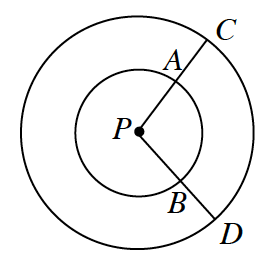### Home > INT2 > Chapter 11 > Lesson 11.1.1 > Problem11-10

11-10.The figure at right shows two concentric circles.

1. What is the relationship between $\overarc { A B }$ and $\overarc { C D }$? How can $\overarc { A B }$ be mapped to $\overarc { C D }$ using a transformation?

They are both intercepted by $\angle CPD$.
Dilate $\overarc{AB}$ from $P$ to get $\overarc { C D }$.

2. Which has greater measure, $\overarc { A B }$ or $\overarc { C D }$? Which has greater length? Explain.

Both arcs have the same central angle but are part of different circles.

3. If $m∠P = 60°$ and $PD = 14$, what is the length of $\overarc { C D }$? Show all work.

$\frac{θ}{360°}(\text{circumference})=\text{arc length}$